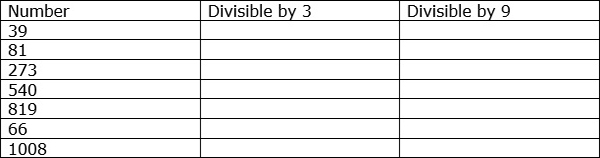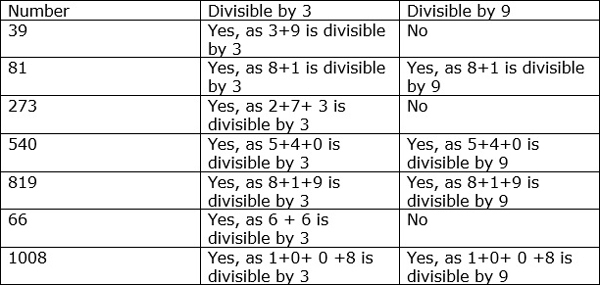# Divisibility Rules for 3 and 9

If the sum of the digits of a number is divisible by 3, then the number is divisible by 3.

Some examples of numbers divisible by 3 are as follows.

• The number 85203 is divisible by 3 because the sum of its digits (8 + 5 + 2 + 0 + 3 = 18) is divisible by 3.
• The number 79154 is not divisible by 3 because the sum of its digits (7 + 9 + 1 + 5 + 4 = 26) is not divisible by 3.

If the sum of the digits of a number is divisible by 9, then the number is divisible by 9.

Some examples of numbers divisible by 9 are as follows.

• The number 51984 is divisible by 9 because the sum of its digits (5+ 1 + 9 + 8 + 4 = 27) is divisible by 9.
• The number 91403 is not divisible by 9 because the sum of its digits (9 + 1 + 4 + 0 + 3 = 17) is not divisible by 9.

Find the divisibility of the numbers in following table### Solution